# JEE Main 2020 (Shift 1-4th Sept) Physics Paper With Solutions

JEE Main is an examination conducted for admission into some of the prestigious institutions in India. The examination is considered to be one of the toughest, which a candidate can crack only by preparing smartly. One of the ways to prepare for the examination is by practising previous years’ questions papers. Solving previous years’ question papers is helpful to increase problem-solving speed and to understand the pattern of the question paper. Click on the link below to download the Physics 2020 Shift 1 paper.

### September 4 Shift 1 - Physics

1. Starting from the origin at time t = 0, with initial velocity

$$5\hat{j}ms^{-1}$$
, a particle moves in the x-y plane with a constant acceleration of
$$(10\hat{i}+4\hat{j})ms^{-2}$$
. At time t, its coordinates are(20 m,y0 m). The values of t and y0 are, respectively:

1. 1) 5s and 25 m
2. 2) 2s and 18 m
3. 3) 2s and 24 m
4. 4) 4s and 52 m

Solution:

1. Equation of motion gives us

$$y = u_{y}t + \frac{1}{2}a_{y}t^2$$

Here uy= 5ms–1, ux= 0 ms–1, ax= 10ms–2, ay= 4ms–2

y = 5t+2t2

and x = 0 +(1/2)(10) (t2) = 20

t = 2s

y = 10 + 8 = 18m

2. A small bar magnet placed with its axis at 30° with an external field of 0.06 T experiences a torque of 0.018 Nm. The minimum work required to rotate it from its stable to unstable equilibrium position is:

1. 1) 7.2 × 10–2 J
2. 2) 6.4 × 10–2 J
3. 3) 9.2 × 10–3 J
4. 4) 11.7 × 10–3 J

Solution:

1. Τ = MB sin 30º

0.018 = MB(1/2)

MB = 0.036

w = ∆U = |MB cos00 —MB cos 1800| = 2MB = 7.2 × 10–2 J

3. Choose the correct option relating wave lengths of different parts of electromagnetic wave spectrum:

1. 1) λ radio waves > λ micro waves > λ visible > λ x-rays
2. 2) λ visible > λ x-rays > λ radio waves > λ micro waves
3. 3) λ visible < λ micro waves < λ radio waves < λ x-rays
4. 4) λ x-rays < λ micro waves < λ radio waves < λ visible

Solution:

By property of electromagnetic wave spectrum.

4. On the x-axis and at a distance x from the origin, the gravitational field due a mass distribution is given by

$$\frac{Ax}{(x^{2}+a^{2})^{\frac{3}{2}}}$$
in the x-direction. The magnitude of gravitational potential on the x-axis at a distance x, taking its value to be zero at infinity, is:

1. 1)
$${A}{(x^{2}+a^{2})^{\frac{3}{2}}}$$
2. 2)
$$\frac{Ax}{(x^{2}+a^{2})^{\frac{1}{2}}}$$
3. 3)
$${A}{(x^{2}+a^{2})^{\frac{1}{2}}}$$
4. 4)
$$\frac{A}{(x^{2}+a^{2})^{\frac{3}{2}}}$$

Solution: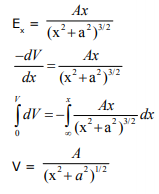5. A small bar magnet is moved through a coil at constant speed from one end to the other. Which of the following series of observations will be seen on the galvanometer G attached across the coil?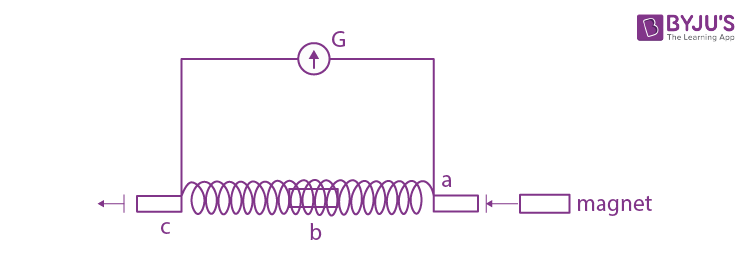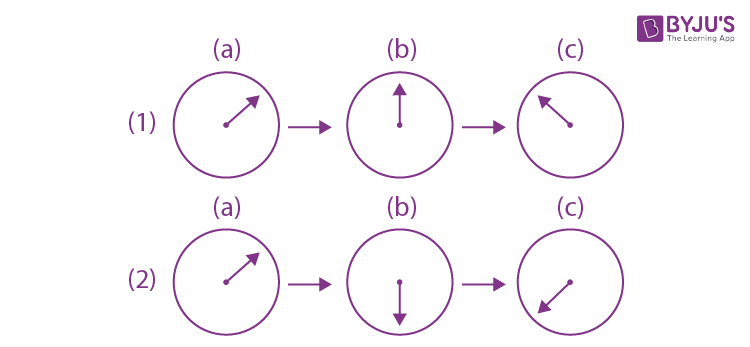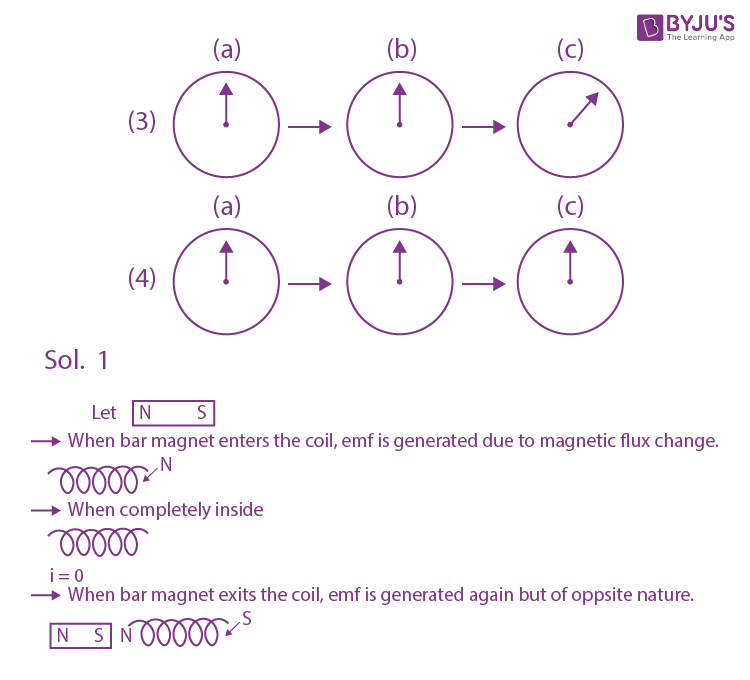Solution:

6. A battery of 3.0V is connected to a resistor dissipating 0.5 W of power. If the terminal voltage of the battery is 2.5V, the power dissipated within the internal resistance is:

1. 1) 0.072 W
2. 2) 0.10 W
3. 3) 0.125 W
4. 4) 0.50 W

Solution:PR = 0.5 W

⇒ i2R = 0.5 W

Also, V = E – ir

2.5 = 3 – ir

⇒ir = 0.5

Power dissipated across 'r' " Pr = i2r

Now iR = 2.5

ir = 0.5

On dividing : R / r = 5

$$Now, \frac{P_{R}}{P_{r}} = \frac{i^{2}R}{i^{2}r}\Rightarrow \frac{P_{R}}{P_{r}}\frac{R}{r}\Rightarrow \frac{P_{R}}{P_{r}} = 5$$

$$P_{r} = \frac{P_{R}}{5}$$

$$P_{r} = \frac{0.50}{5} = 0.10\: W$$

7. Two charged thin infinite plane sheets of uniform surface charge density σ + and σ , where| σ +| > |σ |, intersects at right angle. Which of the following best represents the electric field lines for this system?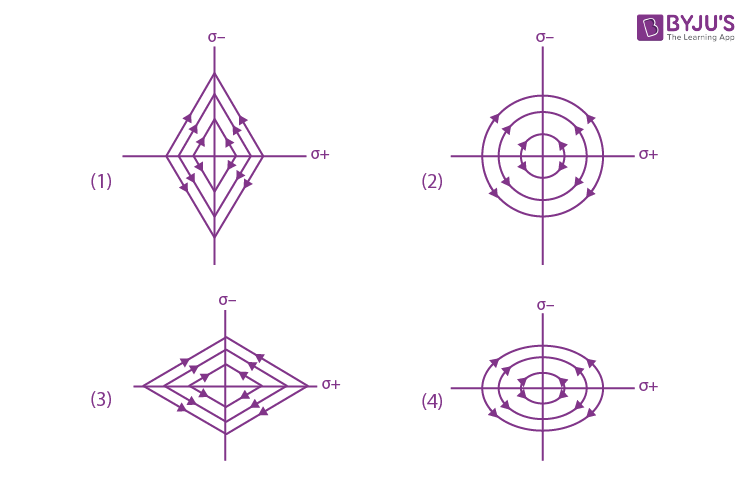Solution:

Let us choose points A,B,C,D as shown to understand the direction of net electric field

to get a better picture.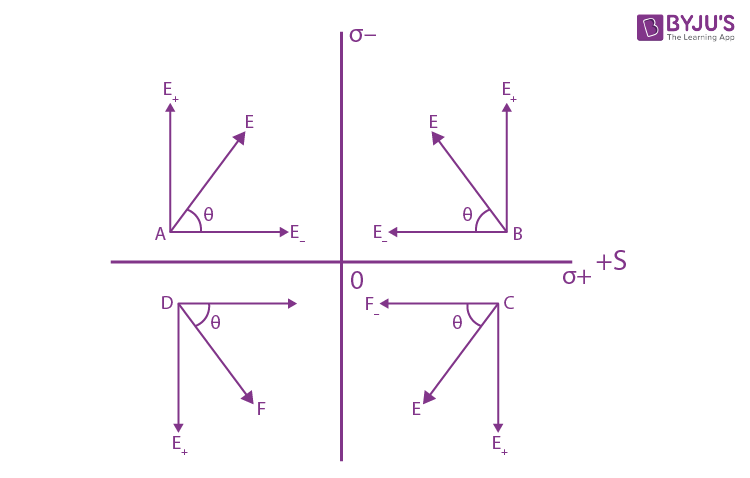8. An air bubble of radius 1 cm in water has an upward acceleration 9.8 cm s–2. The density of water is 1 gm cm–3 and water offers negligible drag force on the bubble. The mass of the bubble is (g = 980 cm/s2).

1. 1) 1.52 gm
2. 2) 4.51 gm
3. 3) 3.15 gm
4. 4) 4.15 gm

Solution: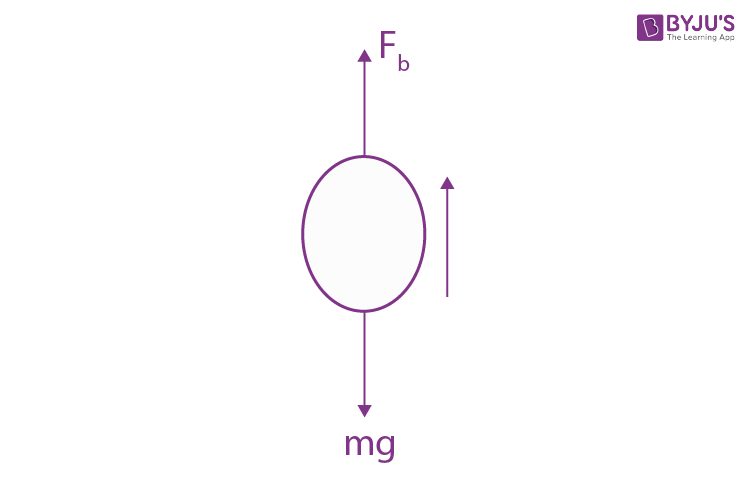Fb–mg = ma

m=Fb/(g+ a)

m=V ρwg/(g+a)

$$m=\frac{(4/3)\pi r^{3}.\rho _{w}.g}{(g+a)}=4.15 gram$$

9. A wire A, bent in the shape of an arc of a circle, carrying a current of 2A and having radius 2 cm and another wire B, also bent in the shape of arc of a circle, carrying a current of 3 A and having radius of 4 cm, are placed as shown in the figure. The ratio of the magnetic field due to the wires A and B at the common centre O is: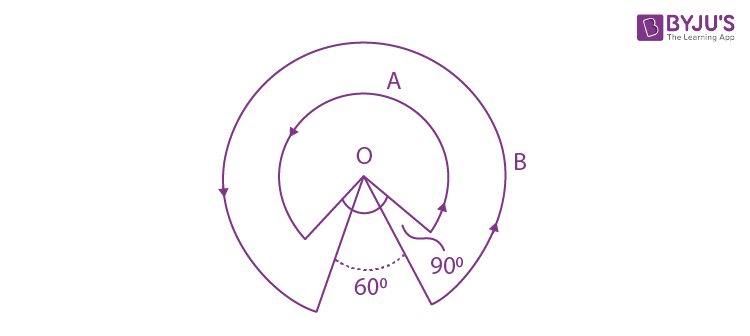1. 1) 2 : 5
2. 2) 6 : 5
3. 3) 6 : 4
4. 4) 4 : 6

Solution:

$$B_{A}=\frac{\mu(2)\left [ \frac{3\pi }{2} \right ] }{2a(2\pi )}=3\mu/4a$$

$$B_{B}=\frac{\mu(3)\left [ \frac{5\pi }{3} \right ] }{2(2a)(2\pi )}=5\mu/8a$$

$$\frac{B_{A}}{B_{B}}= \frac{3\mu }{4a}\times \frac{8a}{5\mu }=6:5$$

10. Particle A of mass mA=m/2 moving along the x-axis with velocity v0 collides elastically with another particle B at rest having mass mB=m/3. If both particles move along the x-axis after the collision, the change ∆ λ in de-Broglie wavelength of particle A, in terms of its de-Broglie wavelength (λ0) before collision is:

1. 1) ∆λ = (5/2)λ0
2. 2) ∆λ = 2λ0
3. 3) ∆λ = 4λ0
4. 4) ∆λ = (3/2)λ0

Solution: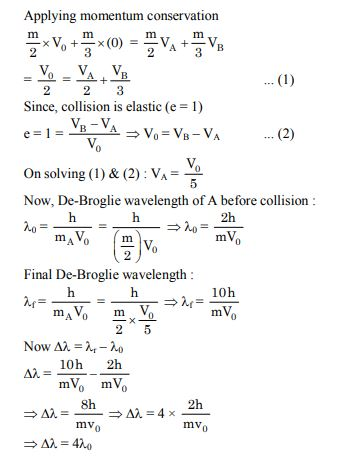11. Blocks of masses m, 2m, 4m and 8m are arranged in a line on a frictionless floor. Another block of mass m, moving with speed v along the same line (see figure) collides with mass m in perfectly inelastic manner. All the subsequent collisions are also perfectly inelastic. By the time the last block of mass 8m starts moving the total energy loss is p% of the original energy. Value of 'p' is close to: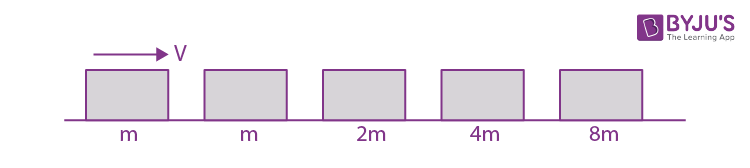1. 1) 94
2. 2) 87
3. 3) 37
4. 4) 77

Solution:All collisions are perfectly inelastic, so after the final collision, all blocks are moving together. So let the final velocity be v, so on applying momentum conservation : mv = 16 m v v = v/16

Now initial energy E1 = ½ mv2

Final energy:

Ef = ½ x 16m x (v / 16)2 ⇒ Ef = ½ m x v2 / 16

Energy loss : Ei - Ef

⇒ ½ mv2 - ½ m x v2 / 16

$$\Rightarrow \frac{1}{2}mv^{2}\left [ 1 - \frac{1}{16} \right ] \Rightarrow \frac{1}{2}mv^{2}\left [ \frac{15}{16} \right ]$$

$$% p = \frac{Energy \: Loss}{Original\: Energy}\times 100$$

$$\Rightarrow \frac{\frac{1}{2}mv^{2}\left [ \frac{15}{16} \right ]}{\frac{1}{2}mv^{2}} \times 100 = 93.75 %$$

⇒ Value of P is quite near to 94.

12. Given figure shows few data points in a photo-electric effect experiment for a certain metal. The minimum energy for ejection of electron from its surface is: (Planck’s constant h = 6.62 × 10–34 J.s)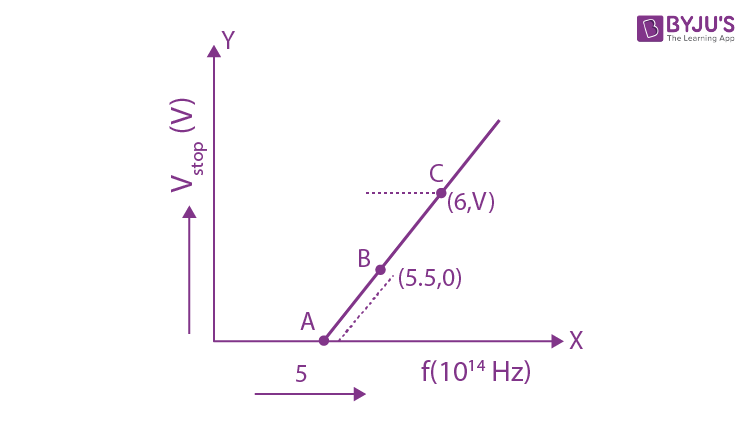1. 1) 2.10 eV
2. 2) 2.27 eV
3. 3) 2.59 eV
4. 4) 1.93 eV

Solution: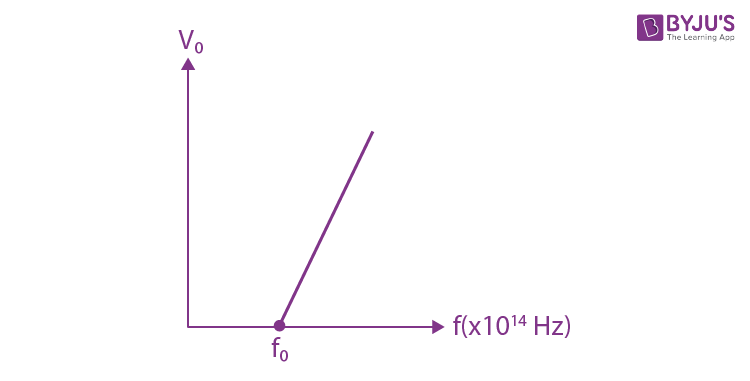Threshold energy = φ = hf0, Here f0 = 5.5 × 1014Hz

φ = hf0

= 6.62 × 10–34 × 5.5 × 1014

= 36.41 × 10–20J = 2.27 eV

13. The specific heat of water = 4200 J kg–1K–1 and the latent heat of ice = 3.4 × 105 J kg-1.100 grams of ice at 0°C is placed in 200 g of water at 25°C. The amount of ice that will melt as the temperature of water reaches 0°C is close to (in grams):

1. 1) 63.8
2. 2) 64.6
3. 3) 61.7
4. 4) 69.3

Solution:

Heat loss by water when it cools down to 00 C is,

Q = mws∆θ = (200/1000).(4200) (25) = 21000 J

Which is less than the amount of heat (mLf) required to melt ice completely.

∆miL = 21000 J

To find the amount of ice melt (∆mi), take

∆mi =[21000/(3.4x105)] x 103 gm = 61.7 grams

14. A beam of plane polarised light of large cross-sectional area and uniform intensity of 3.3 Wm–2 falls normally on a polariser (cross sectional area 3 × 10–4 m2) which rotates about its axis with an angular speed of 31.4 rad/s. The energy of light passing through the polariser per revolution is close to:

1. 1) 1.0 × 10–4 J
2. 2) 1.0 × 10–5 J
3. 3) 5.0 × 10–4 J
4. 4) 1.5 × 10–4 J

Solution:

From Malus’s law

p = p0cos2ωt

here, p0 and p are incident and transmitted intensity respectively.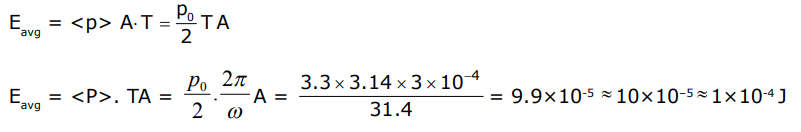15. For a transverse wave travelling along a straight line, the distance between two peaks (crests) is 5m, while the distance between one crest and one trough is 1.5m. The possible wavelengths (in m) of the waves are:

1. 1) 1, 3, 5,.....
2. 2) 1, 2, 3,.....
3. 3)
$$\frac{1}{2},\frac{1}{4},\frac{1}{6}$$
.....
4. 4)
$$\frac{1}{1},\frac{1}{3},\frac{1}{5}.....$$

Solution:

Given trough to crest distance

1.5 = (2n1+ 1) λ/2 ...(1)

and crest to crest distance is

5 = n2λ...(2)

n1 & n2 are integer

n1 = 1, n2 = 5 → λ = 1

n1 = 2, n2 is not integer

n1 = 3, n2 is not integer

n1 = 4, n2 = 15, λ = 1/3

n1 = 7, n2 = 25, λ = 1/5

16. Match the CP/CV ratio for ideal gases with different type of molecules: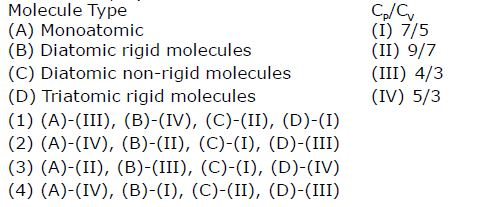Solution:

γ = Cp/Cv

γA = 1 + (2/3) = 5/3

γB = 1 + (2/5) = 7/5

γC = 1 + (2/7) = 9/7

γD = 1 + (2/6) = 4/3

17. Two points charges 4q and –q are fixed on the x-axis at x = -(d/2) and x = (d/2), respectively. If a third point charge 'q' is taken from the origin to x = d along the semicircle as shown in the figure, the energy of the charge will: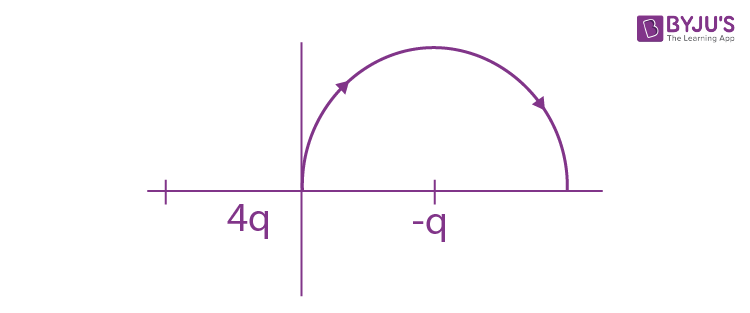1. 1) decrease by
$$\frac{q^{2}}{4\pi \epsilon _{0}d}$$
2. 2) decrease by
$$\frac{4q^{2}}{3\pi \epsilon _{0}d}$$
3. 3) increase by
$$\frac{3q^{2}}{4\pi \epsilon _{0}d}$$
4. 4) increase by
$$\frac{2q^{2}}{3\pi \epsilon _{0}d}$$

Solution: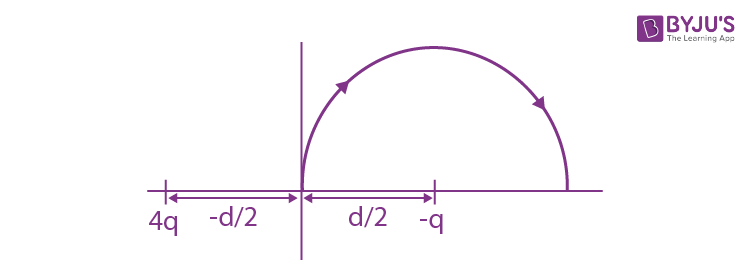Initial and final potential energy are,

$$\mathrm{U}_{\mathrm{i}}=\frac{1}{4 \pi \varepsilon_{0}}\left[\frac{4 \mathrm{q}^{2}}{\left(\frac{\mathrm{d}}{2}\right)}-\frac{\mathrm{q}^{2}}{\left(\frac{\mathrm{d}}{2}\right)}\right]$$

$$\mathrm{U}_{\mathrm{f}}=\frac{1}{4 \pi \varepsilon_{0}}\left[\frac{4 \mathrm{q}^{2}}{\left(\frac{\mathrm{3d}}{2}\right)}-\frac{\mathrm{q}^{2}}{\left(\frac{\mathrm{d}}{2}\right)}\right]$$

$$\mathrm{U}_{\mathrm{f}}-\mathrm{U}_{\mathrm{i}}=\Delta \mathrm{U}=\frac{1}{4 \pi \varepsilon_{0}} \cdot \frac{4 q \cdot q}{(3 \mathrm{d} / 2)}-\frac{1}{4 \pi \varepsilon_{0}} \cdot \frac{4 q \cdot q}{(d / 2)}$$

$$=\frac{4 q^{2}}{4 \pi \varepsilon_{0}}\left(\frac{2}{d}\right)\left(-\frac{2}{3}\right)$$

$$=(-) \frac{4 q^{2}}{3 \pi \varepsilon_{0} \cdot d}$$

= decrease by

$$\frac{4q^{2}}{3\pi \epsilon _{0}d}$$

18. A Tennis ball is released from a height h and after freely falling on a wooden floor it rebounds and reaches height h/2. The velocity versus height of the ball during its motion may be represented graphically by: (graphs are drawn schematically and are not to scale)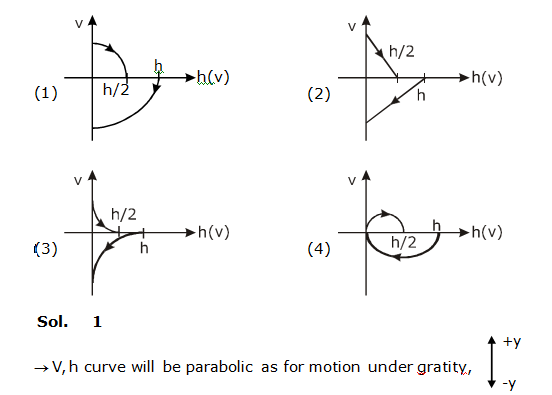Solution:

1. v2 = u2 + 2gh downward velocity is negative and upward is positive when ball is coming down graph will be in IV quadrant i.e. v is –ve and when going up graph will be in I quadrant i.e. v is +ve.

19. Dimensional formula for thermal conductivity is (here K denotes the temperature):

1. 1) MLT–3 K–1
2. 2) MLT–2 K–2
3. 3) MLT–2 K
4. 4) MLT–3 K

Solution:

Thermal current during steady state conduction of heat along a rod is,

(dQ /dT) =- KA(dt/dx)

Using dimensional analysis we get [M1L1T-3 K-1]

20. Take the breakdown voltage of the zener diode used in the given circuit as 6V. For the input voltage shown in figure below, the time variation of the output voltage is : (Graphs drawn are schematic and not to scale)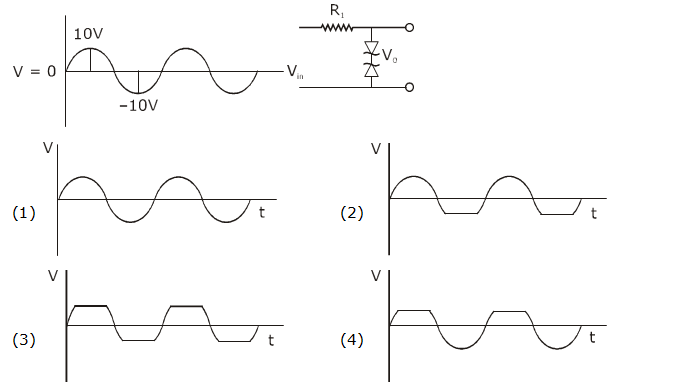Solution: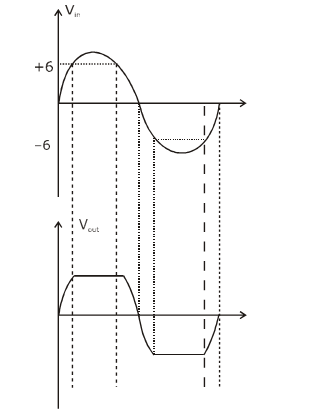21. In the line spectra of hydrogen atoms, difference between the largest and the shortest wavelengths of the Lyman series is 304Å. The corresponding difference for the Paschen series in Å is : ___________.

Solution:

For shortest wave length in Lyman, we have

$$\frac{1}{\lambda }=R\left [ 1 \right ]$$
(i.e. n= ∞ to n =1)

For longest wave length in Lyman

$$\frac{1}{\lambda^{'} }=R\left [ 1 -\frac{1}{4}\right ]=\frac{3R}{4}$$

In Paschen series, for shortest wave length

$$\frac{1}{\lambda^{s} }=R\left [ \frac{1}{3^{2}} -\frac{1}{ \infty^{2} }\right ]$$

$$\frac{1}{\lambda^{s} }=R\left [ \frac{1}{3^{2}}\right ]=\frac{R}{9}$$

And for longest wave length

$$\frac{1}{\lambda_{l} }=R\left [ \frac{1}{3^{2}}-\frac{1}{4^{2}}\right ]=\frac{7R}{144}$$

Now, taking ratio we get

$$(\lambda _{l}-\lambda _{s})$$
= 10553 Å

22. A closed vessel contains 0.1 mole of a monoatomic ideal gas at 200 K. If 0.05 mole of the same gas at 400 K is added to it, the final equilibrium temperature (in K) of the gas in the vessel will be close to _______.

Solution:

As work done on gas and heat supplied to the gas are zero, the total internal energy of gases remain same;

u1 + u2 = u1’ + u2’

(0.1) Cv (200) + (0.05) Cv (400) = (0.15) Cv T

T = 800 / 3 k = 266.67 k

23. ABC is a plane lamina of the shape of an equilateral triangle. D, E are mid points of AB, AC and G is the centroid of the lamina. Moment of inertia of the lamina about an axis passing through G and perpendicular to the plane ABC is I0. If part ADE is removed, the moment of inertia of the remaining part about the same axis is NI0 /16 where N is an integer. Value of N is _____________.Solution:

If m is mass of lamina and l is its side length, then moment of inertia of lamina about an axis passing through G and perpendicular to plane is I0.Let I0 = kml2

$$I_{DEF}=K\left ( \frac{m}{4} \right )\left ( \frac{l}{2} \right )^{2}=\left ( \frac{I_{0}}{16} \right )$$

and IADE = IBDE = IEFC = I (say)

Then,

$$3I = I_{0}-\frac{I_{0}}{16}=\frac{15I_{0}}{16}$$

$$\Rightarrow I =\frac{5I_{0}}{16}$$

$$I_{remaining}=2I+\frac{I_{0}}{16}=\frac{11I_{0}}{16}$$

24. In a compound microscope, the magnified virtual image is formed at a distance of 25 cm from the eye-piece. The focal length of its objective lens is 1 cm. If the magnification is 100 and the tube length of the microscope is 20 cm, then the focal length of the eyepiece lens (in cm) is __________.

Solution:

L = 20, f0 = 1cm, M = 100

$$M=\frac{v_{0}}{u_{0}}\left ( 1+\frac{D}{f_{e}} \right )$$

$$M=\frac{L}{f_{0}}\left ( 1+\frac{D}{f_{e}} \right )$$
(since vo ≈L, u0≈f0)

$$\Rightarrow 100 = \left [ \frac{20}{1} \right ]\left [ 1+\frac{25}{f_{e}} \right ]$$

on solving we get

f e = 6.25 cm

25. A circular disc of mass M and radius R is rotating about its axis with angular speed ω1 . If another stationary disc having radius R/2 and same mass M is droped co-axially on to the rotating disc. Gradually both discs attain constant angular speed ω2. The energy lost in the process is p% of the initial energy. Value of p is __________.

Solution:

If ωf = Iiωi

$$I_{i}=\frac{MR^{2}}{2}$$

$$I_{f}=\frac{MR^{2}}{2}+\frac{M(R/2)^{2}}{2}$$

$$I_{f}=\frac{5}{4}\frac{MR^{2}}{2}$$

$$\left [ \frac{MR^{2}}{2}+\frac{M(R/2)^{2}}{2} \right ]\omega '=\left [ \frac{MR^{2}}{2} \right ]\omega$$

$$\left [ \frac{MR^{2}}{2}\left ( \frac{5}{4} \right ) \right ]\omega '=\left [ \frac{MR^{2}}{2} \right ]\omega$$

$$\omega '=\frac{4}{5}\omega$$

$$loss of K.E = \frac{Loss}{K_{i}}\times 100=\frac{\omega ^{2}-\omega '^{2}(\frac{5}{4})}{\omega ^{2}}\times 100$$

$$=\frac{\omega ^{2}-\frac{16}{25}\omega ^{2}(\frac{5}{4})}{\omega ^{2}}=\left [ 1-\frac{80}{100} \right ]\times 100=20%$$

### JEE Main 2020 Physics Paper With Solutions Shift 1 September 4